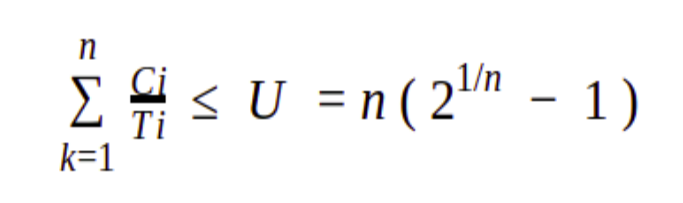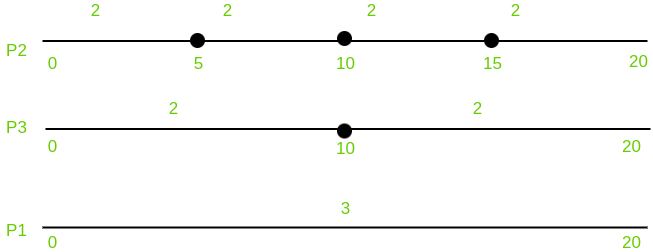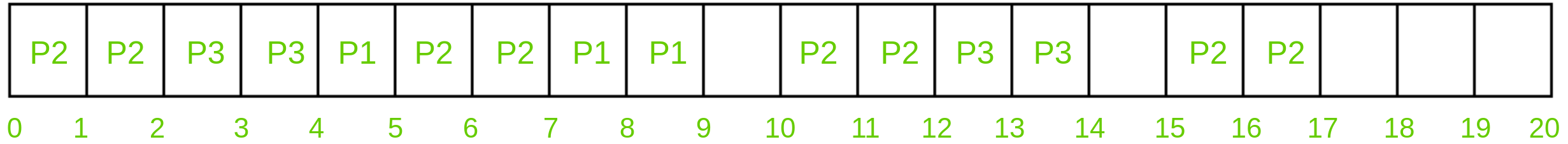# Rate-monotonic scheduling

Rate monotonic scheduling is a priority algorithm that belongs to the static priority scheduling category of Real Time Operating Systems. It is preemptive in nature. The priority is decided according to the cycle time of the processes that are involved. If the process has a small job duration, then it has the highest priority. Thus if a process with highest priority starts execution, it will preempt the other running processes. The priority of a process is inversely proportional to the period it will run for.

A set of processes can be scheduled only if they satisfy the following equation :Where n is the number of processes in the process set, Ci is the computation time of the process, Ti is the Time period for the process to run and U is the processor utilization.

Example:
An example to understand the working of Rate monotonic scheduling algorithm.

Processes Execution Time (C) Time period (T)
P1 3 20
P2 2 5
P3 2 10
```n( 2^1/n - 1 ) = 3 ( 2^1/3 - 1 ) = 0.7977

U = 3/20 + 2/5 + 2/10 = 0.75 ```

It is less than 1 or 100% utilization. The combined utilization of three processes is less than the threshold of these processes that means the above set of processes are schedulable and thus satisfies the above equation of the algorithm.

1. Scheduling time –
For calculating the Scheduling time of algorithm we have to take the LCM of the Time period of all the processes. LCM ( 20, 5, 10 ) of the above example is 20. Thus we can schedule it by 20 time units.

2. Priority –
As discussed above, the priority will be the highest for the process which has the least running time period. Thus P2 will have the highest priority, after that P3 and lastly P1.

`P2 > P3 > P1 `
3. Representation and flow –Above figure says that, Process P2 will execute two times for every 5 time units, Process P3 will execute two times for every 10 time units and Process P1 will execute three times in 20 time units. This has to be kept in mind for understanding the entire execution of the algorithm below.Process P2 will run first for 2 time units because it has the highest priority. After completing its two units, P3 will get the chance and thus it will run for 2 time units.

As we know that process P2 will run 2 times in the interval of 5 time units and process P3 will run 2 times in the interval of 10 time units, they have fulfilled the criteria and thus now process P1 which has the least priority will get the chance and it will run for 1 time. And here the interval of five time units have completed. Because of its priority P2 will preempt P1 and thus will run 2 times. As P3 have completed its 2 time units for its interval of 10 time units, P1 will get chance and it will run for the remaining 2 times, completing its execution which was thrice in 20 time units.

Now 9-10 interval remains idle as no process needs it. At 10 time units, process P2 will run for 2 times completing its criteria for the third interval ( 10-15 ). Process P3 will now run for two times completing its execution. Interval 14-15 will again remain idle for the same reason mentioned above. At 15 time unit, process P2 will execute for two times completing its execution.This is how the rate monotonic scheduling works.

Conditions :
The analysis of Rate monotonic scheduling assumes few properties that every process should possess. They are :

1. Processes involved should not share the resources with other processes.
2. Deadlines must be similar to the time periods. Deadlines are deterministic.
3. Process running with highest priority that needs to run, will preempt all the other processes.
4. Priorities must be assigned to all the processes according to the protocol of Rate monotonic scheduling.

1. It is easy to implement.
2. If any static priority assignment algorithm can meet the deadlines then rate monotonic scheduling can also do the same. It is optimal.
3. It consists of calculated copy of the time periods unlike other time-sharing algorithms as Round robin which neglects the scheduling needs of the processes.

1. It is very difficult to support aperiodic and sporadic tasks under RMA.

Don’t stop now and take your learning to the next level. Learn all the important concepts of Data Structures and Algorithms with the help of the most trusted course: DSA Self Paced. Become industry ready at a student-friendly price.

My Personal Notes arrow_drop_upCheck out this Author's contributed articles.

If you like GeeksforGeeks and would like to contribute, you can also write an article using contribute.geeksforgeeks.org or mail your article to contribute@geeksforgeeks.org. See your article appearing on the GeeksforGeeks main page and help other Geeks.

Please Improve this article if you find anything incorrect by clicking on the "Improve Article" button below.

Improved By : pp_pankaj